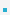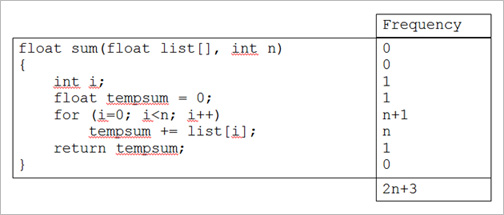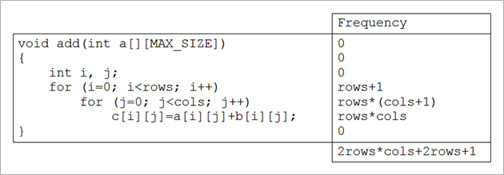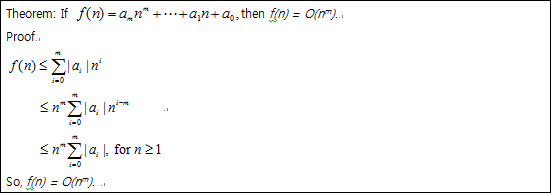1. Basic Concepts

1.1 Allocation and deallocation of memory

 int main() {     int i, *pi;     if ( !(pi = (int *)malloc(sizeof(int))) )     {        fprintf(stderr, “Insufficient memory”);        exit(0);     }     *pi = 3;     printf(“%d\n”, *pi);     free(pi);     return 0; }

1.2 Algorithm SpecificationDefinition: An algorithm is a finite set of instructions that, if followed, accomplishes a particular task.    In addition, all algorithms must satisfy the following criteria.
1) Input. There are zero or more quantities that are externally supplied.
2) Output. At least one quantity is produced.
3) Definiteness. Each instruction is clear and unambiguous.
4) Finiteness. If we trace out the instructions of an algorithm, then for all cases, the algorithm     terminates after a finite number of steps.
5) Effectiveness. Every instruction must be basic enough to be carried out, in principle, by a person     using only pencil and paper. It is not enough that each operation be definite as in 3); it also must     be feasible.In computational theory, one distinguishes between an algorithm and a program, the latter of which    does not have to satisfy the fourth condition. For example, we can think of an operating system that    continues in a wait loop until more jobs are entered.

Example[Selection sort] Suppose we must devise a program that sorts a set of n ≥ 1 integers.STEP 1. From those integers that are currently unsorted, find the smallest and place it next in the    sorted list.STEP 2. Write it partially in C and partially in English

 for (i=0; i

 #include #include // rand() #define SWAP(x, y, t) ((t)=(x), (x)=(y), (y)=(t)) void sort(int list[], int n); int main(void) {     int i, list;     for (i=0; i<100; i++) list[i] = rand() % 1000;     sort(list, i);     for (i=0; i<100; i++) printf("%d\n", list[i]);     return 0; } void sort(int list[], int n) {     int i, j, min, temp;     for (i=0; i

1.3 Performance AnalysisOne of the goals of this course to develop your skills for making evaluative judgments about   programs.
1) Does the program meet the original specifications of the task?
2) Doest it work correctly?
3) Doest the program contain documentation that shows how to use it and how it works?
4) Doest the program effectively use functions to create logical units?
5) Is the program’s code readable?
6) Does the program efficiently use primary and secondary storage?
7) Is the program’s running time acceptable for the task?These criteria focus on performance evaluation, we can loosely divide into two distinct fields:
space complexity and time complexity.

1.3.1 Space ComplexityThe amount of memory that it needs to run to completion.Fixed space requirement
– Space requirements that do not depend on the number and size of the program’s input and    outputs.
– Include the instruction space, space for simple variables, fixed-size structured variables (such as    structs), and constants.Variable space requirement
– The space needed by structured variables whose size depends on the particular instance, I, for the    problem being solved.
– Include the additional space required when a function uses recursion.The total space requirement = fixed + variable
S(P) = c + SP(I)
– c: constant representing the fixed space requirements.
SP(I) : the variable space requirement of a program P working on an instance I. It is represented by SP(n) if n is the only instance characteristic of the instance I.

Example: summing a list of numbersIterative function: Ssum(n) = 0
 float sum(float list[], int n) {     int i; float tempsum = 0;     for (i=0; iRecursive function: Srsum(n) = 12*n bytes
 float rsum(float list[], int n) {     if (n) return rsum(list, n-1) + list[n-1];     return 0; }

 Type Name Number of bytes array pointer list[ ] 4 integer n 4 return address 4 Total per recursive call 12

1.3.2 Time ComplexityThe amount of computer time that it needs to run to completion.The time, T(P) taken by a program, P,
T(P) = compile time + TP(I)
– The compile time is similar to the fixed space component.
TP(I): program run (or execution) timeMachine-dependent estimate of TP(I)
– Not easy task because it
– Letting n denote the instance characteristic, we might express
TP(n) = caADD(n) + csSUB(n) + clLDA(n) + cstSTA(n)
– where are constants that refer to the time needed to perform each operation, and ADD, SUB, LDA,    STA are number of additions, subtractions, loads, and stores that are performed.Machine-independent estimate of TP(I)
– Program step

Program StepDefinition: A program step is a syntactically or semantically meaningful program segment whose    execution time is independent of the instance characteristics.Step count for summingStep count for recursive summingStep count for summing1.4 Asymptotic NotationDefinition: [Big “oh”] f(n) = O(g(n)) (read as “f of n is big oh g of n”) iff (if and only if) there exist    positive constants c and n0 such that f(n) ≤ cg(n) for all n, n ≥ n0.

Example

 3n+2 = O(n) 3n+2 ≤ 4n for all n ≥ 2 100n+6 = O(n) 100n+6 ≤ 101n for all n ≥ 10 10n2+4n+2 = O(n2) 10n2+4n+2 ≤ 11n2 for all n ≥ 5 6*2n+n2 = O(2n) 6*2n+n2 ≤ 7*2n for all n ≥ 4

 O(1) a computing time is constant O(n) linear O(n2) quadratic O(n3) cubic O(2n) exponentialThe seven computing times
– O(1), O(log n), O(n), O(n log n), O(n2), O(n3), O(2n)1.5 Performance MeasurementWe discuss on measuring time.There are actually two different methods for timing events in C, by using C’s standard library accessed    through #include <time.h>Method 1: clock()
– This function gives the amount of processor time, as the built-in type clock_t, that has elapsed since    the program began running.Method 2: time()
– This function returns the time, measured in seconds, as the built-in type time_t.

 Method 1 Method 2 Start timing start = clock(); start = time(NULL); Stop timing stop = clock(); stop = time(NULL); Type returned clock_t time_t Result in seconds duration = ((double)(stop-start))   /CLOCKS_PER_SEC; duration = (double) difftime(stop, start);

Example: timing program for worst-case sortingO(n) vs O(n2)
 #include #define MAX_SIZE 1001 void sort(int list[], int n); int main(void) {     int i, n, step = 10;     int a[MAX_SIZE];     double duration;     clock_t start;     for (n=0; n

 이전페이지 / 2 / 다음페이지